Technical Information Site of Power Supply Design

• TECH INFO
• What capacitor and inductor are the best for a switching power supply? - Capacitor - : In Selecting an Input Capacitor, Focus on the Ripple Current, ESR, and ESL

Ask Direct to Engineers

What capacitor and inductor are the best for a switching power supply?

# In Selecting an Input Capacitor, Focus on the Ripple Current, ESR, and ESL

## Capacitor －Part 3－

Keyword MLCCInput capacitorRipple voltageRipple current ratingESRESLSelf heatingLifetime

– I now understand that the properties of a capacitor change according to the materials and packaging case involved. That said, please tell me when a capacitor is used in an actual switching power supply circuit, in what ways its properties and characteristics will exert specific impact.

Switching power supply circuits require both input and output capacitors. As you may know, each capacitor has different properties in terms of the voltage and current that it can handle. For ease of understanding, I think I should discuss input and output separately. Let me start off with an input capacitor. In a way of recap and as preparatory to what follows, here is a brief rundown of the current that flows through the input capacitor.

The figure below illustrates the circuit for a conceptual synchronous rectifying step-down converter. Looking at VIN, the MOSFET on the nearside represents a high-side switch. When the high-side switch turns on, its current waveform rises almost vertically, producing the same current as the inductor current. When the high-side switch turns off and the low-side switch turns on, the current rapidly falls to zero. The AC component of the current waveform flows into the input capacitor.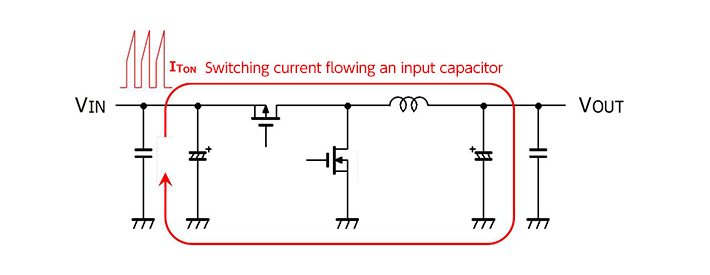The voltage (waveform) generated by the current flowing from the input capacitor takes on different expressions according to differences in the “ESR (equivalent series resistance)”and “ESL (equivalent series inductance)] of the parasitic components that exist in addition to the “capacitance” of the capacitor.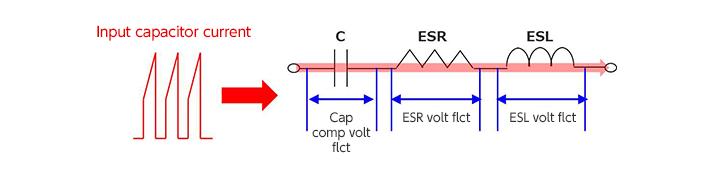– In simple terms, do you mean that both ESR and ESL exert impacts, in addition to the electrostatic capacitance?

Yes, that would be the case. Because this interview affords a valuable opportunity, I’d like to delve into it a little bit more. The impacts of the three elements I mentioned earlier, capacitance, ESR, and ESL, can be expressed in waveforms and equations. To make it easy to see, I have prepared figures.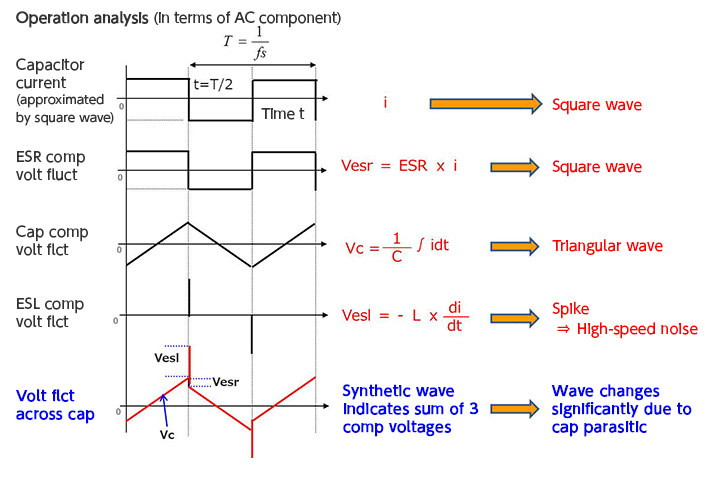The figure, in which the capacitor current is described as square waves, shows what voltages are generated by the various components. First, look at the voltage generated by the ESR. As indicate by the equation, the voltage equals ESR, that is, resistance x current, which produces square waves. The capacitance component is the integral of current and time, turning into triangular waves. The ESL component can be expressed as a derivative; at switching timing, an instantaneous pulse voltage occurs. This can be regarded as high-speed pulse-like noise which is also referred to as a spike. Finally, the voltage fluctuations across the capacitor represent a composite wave which is the sum of these three voltage components.

– I believe that the composite wave of three components must be familiar to most people who have had a chance to evaluate switching power supplies. Would it be acceptable to think that it is simply the sum of the influences of the components?

Basically that would be the case. In fact, that is the important point. If the square wave component is large, for example, clearly the ESR is also large. Also, if the spike is large, we can infer that the ESL should be large. Although the best scenario is one in which both components are zero, because in reality they are non-zero, during the evaluation process you can observe the current and voltage on the input capacitor, and from the waveform that are checked determine the kinds of problems that may have occurred.

Here is data on actual waveforms based on a test circuit. If you would look at the real waveforms, let me explain what differences emerge from tantalum and MLCC.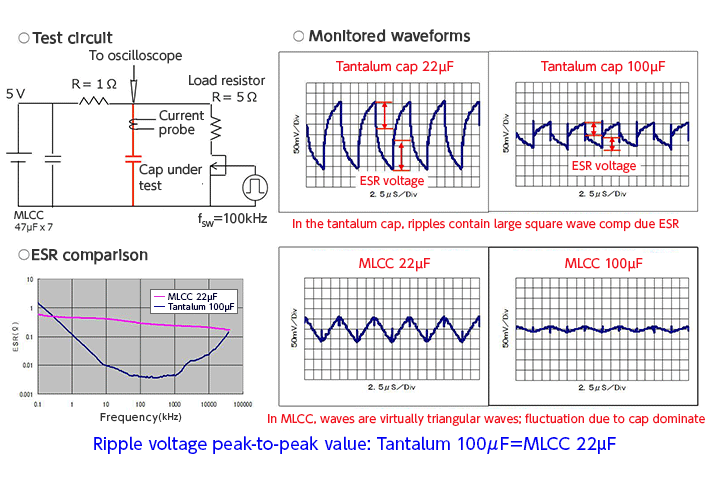First of all, the test circuit is designed to apply 5V/100kHz switching to the capacitor being evaluated, and monitor the voltage and current. The capacitor’s capacitance is of two types: 22 µF and 100 µF. Due to differences in the materials with which they are made, the tantalum capacitor and MLCC have significantly different ESR values. As I mentioned before, this is one of the advantages that the MLCC provides. The graph comparing ESR levels indicates that at 100 kHz the ESR for the MLCC is two orders of magnitude lower, despite the fact that the MLCC has a capacitance which is only one-fifth that of the tantalum capacitor.

First, let us look at the voltage waveform of the 22 µF tantalum. The virtually vertical voltage change part, indicated by the red arrow, represents the square wave component due to the ESR. Due to the large ESR, the ripple voltage that has occurred is also large. At 100 µF, it is clear that the voltage due to the ESR declines to less than half. In other words, the graph shows that the ESR level has fallen to less than one-half. Although some spikes due to ESL can be seen, they do not significantly exceed the overall ripple voltage; consequently, it appears that there are no problems attributable to ESL.

– Now that I have seen the waveform for the lower-side MLCC, let me ask you a question on it first: Do I understand correctly that the reason that the waveform consists of triangular waves and only small spikes are seen is that the because the ESR is extremely small, there are few square wave components due to the ESR, and that because the ESL is also smaller than that in capacitors made of tantalum or functional polymers, the spikes are small, and triangular waves principally due to the capacitance component are visible?

Yes, precisely. Setting the capacitance to 100 µF makes the resulting triangular wave even smaller; thus, it is evident that the ripple voltage is extremely small. What can be stated here is that by using MLCC with small ESR and ESL values, we can clearly reduce the ripple voltage and the spikes. From another angle, if you would look at the waveform graph again, the 100 µF tantalum and the 22 µF MLCCs produce virtually the same ripple voltage. In other words, to achieve a given level of input ripple voltage, the MLCC results in a smaller capacitance while at the same time providing the benefit of being smaller in size.

One more thing: I have data comparing the input ripples between a functional polymer capacitor and a MLCC, using a power supply IC evaluation board. Please take a look for your reference. Note that the functional polymer material has an 82 µF capacitance, whereas the MLCC, at 30 µF, is less than half that capacitance, and that the functional polymer material exhibits somewhat larger spikes due to ESL. Another point is the size. Two capacitors each are visible to the left of the input capacitor enclosed in red; these are output capacitors. Two MLCCs can be fitted in the space that a functional polymer capacitor would require: a result of the fact that basically the MLCC is small in size, with a further reduction in capacitance.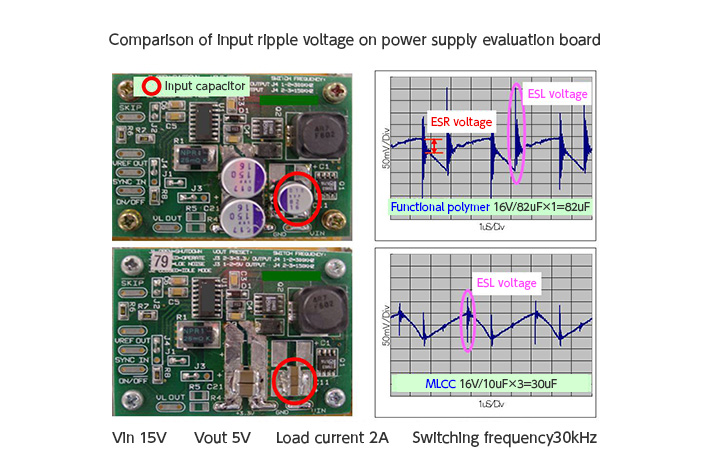– Shifting to another topic, most power supply IC data sheets that we reference state that “in selecting an input capacitor, pay particular attention to ripple current ratings.” Does this have anything to do with the types and properties of the capacitors involved?

The phrase “pay particular attention to ripple current ratings” means that, first of all, capacitors that are capable of accommodating the input ripple current should be used. However, because in input capacitors a ripple current larger than that which is applicable to the output capacitor flows, in this respect in using an input capacitor more care should be exercised with the ripple current rating than would be the case with the output capacitor. Furthermore, ripple current ratings have a bearing on self-heating. Given that the ESR for a capacitor is a resistor component, it generates heat when a ripple current flows in the capacitor. The larger the ESR, the greater the amount of heat generated, as would be expected. In addition, because the ripple current in an input capacitor is large, we should take into consideration the fact that the amount of heat generated can also be large.

– It is often said that in commonly available aluminum electrolyte capacitors the relationship between temperature and lifetime must be examined. Does that also apply to other types of capacitors?

In principle, the same concept applies across the board. Though variable in extent, the fact of the matter is that in any capacitor the higher the temperature the lower the reliability, including lifetime. As an example, for electrolytic capacitors and functional polymer capacitors, in many cases an acceptable current value at 100 kHz is indicated as a ripple current rating condition. We normally design the capacitor using that information as a guide. For MLCCs, in some cases a slightly different condition is indicated. We indicate the condition “Maximum self-heating: 20℃”. (Recently we changed it to 20℃.)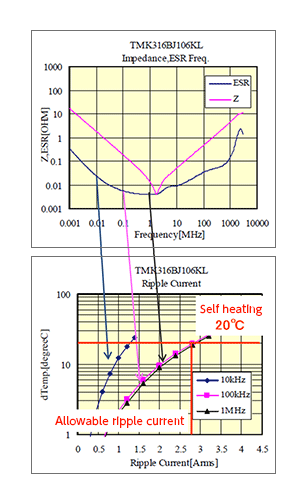As an example, as excerpts from data sheets on a MLCC, let me show you a graph for determining the amounts of self-heating generated and the allowable ripple current that occurs, based upon a switching frequency. The illustration also provides a correspondence with a frequency-ESR graph.

The lower graph showing an allowable ripple current provides a temperature rise curves with respect to the ripple current as a function of switching frequencies 10 kHz, 100 kHz, and 1 MHz. For example, at a 1MHz switching frequency, in order to comply with the “self-heating temperature under 20℃” rule, it is evident that a ripple current which is equal to 2.8A at which the 10℃ and 1MHz curves intersect can be tolerated.

Also, from the above frequency-ESR graph, the ESR values for the three frequencies indicated as conditions can be read. In particular, in the 100 kHz to several MHz range that is used in DC/DC converters, the ESR provides properties that are advantageous in minimizing heat generation in the lowest range. By contrast, under a 10 kHz condition that increases the ESR to some extent, it can be seen that the allowable ripple current is minimized. Obviously, the allowable ripple current is closely related to the ESR, and the MLCC offering small ESR values also provides advantages from a heat generation standpoint.

As you may know, the input ripple current for a power supply circuit can be determined by means of an equation. Taking a step-down converter as an example, I believe that data sheets provide the following type of equation: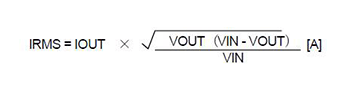The value calculated according to this equation should be within the tolerance shown in the graph.

## Power Supply Design Technical Materials Free DownloadThis website uses cookies.

By continuing to browse this website without changing your web-browser cookie settings, you are agreeing to our use of cookies.